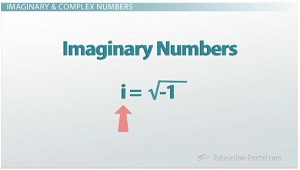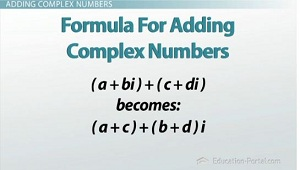# How to Add, Subtract and Multiply Complex Numbers

An error occurred trying to load this video.

Try refreshing the page, or contact customer support.

Coming up next: How to Divide Complex Numbers

### You're on a roll. Keep up the good work!

Replay
Your next lesson will play in 10 seconds
• 0:05 Imaginary and Complex Numbers
• 1:23 Adding Complex Numbers
• 2:12 Subtracting Complex Numbers
• 2:55 Multiplying Complex Numbers
• 5:27 Lesson Summary

Want to watch this again later?

Timeline
Autoplay
Autoplay
Speed

#### Recommended Lessons and Courses for You

Lesson Transcript
Instructor: Luke Winspur

Luke has taught high school algebra and geometry, college calculus, and has a master's degree in education.

Knowing that complex numbers exist is the first step. But that knowledge alone won't help you do much with them. Learn the basics of complex number addition, subtraction and multiplication here!

## Imaginary and Complex Numbers

Imaginary and complex numbers might be the most abstract topic you'll be exposed to in an algebra class. Because they don't actually exist and you just need to imagine that they do, a lot of students struggle with them. But if you can let go and accept that we can still do math with them, it turns out that there isn't anything too tricky about working with them.

But before we get into that, let's quickly review what a complex number is. Any time we take the square root of a negative number, we get an imaginary number. When this happens, we use i to represent the square root of negative 1. This means that i^2 is just regular old -1. It turns back into a real number!When we take an imaginary number and add a real number to it, we end up with a complex number often denoted by a+bi, where a represents the real and b the imaginary portion of the number.

Okay, so how do we do the basic operations on these numbers? This is where it gets nice. Although division will require you to learn a new skill, addition, subtraction and multiplication of complex numbers will all come down to things you already know how to do. As long as you can combine like terms or multiply binomials with FOIL, you're good to go!

## Adding Complex Numbers

What do I mean? Well, for example, adding the two complex numbers (3 - 2i) and (-5 - 4i) is as easy as adding 3 and -5 to get -2, then -2i and -4i to get -6i. This makes our answer -2 - 6i. That's it!

You wouldn't even really have to know that we're adding complex numbers to do this problem. It's all just combining like terms. First combine the real parts, then the imaginary parts, and you're done.

If you like keeping it simple and just thinking about it this way, that's cool. But the formula for adding complex numbers is basically another way of writing what I just said, but with math instead of words. Adding the complex numbers a+bi and c+di gives us an answer of (a+c)+(b+d)i.## Subtracting Complex Numbers

Subtraction is basically the same, but it does require you to be careful with your negative signs. Let's try the example (-2 + 4i) - (3 - i). Combining the real parts looks like -2 - 3=-5, while the negatives will make the imaginary parts a little bit trickier. The first imaginary term 4i minus the second one -i gives us 5i for our imaginary term. Remember that subtracting a negative turns into adding a positive. Therefore our answer is -5+5i.

If you prefer, the formula for subtraction of complex numbers looks like this: (a+bi) - (c+di)=(a - c)+(b - d)i.

## Multiplying Complex Numbers

On to multiplication. This will again rely upon an older skill, the multiplication of binomials. Whether you use FOIL, the area method, the distributive property or one of the other many ways to do it, this is the process of multiplying two terms like (x - 5)(x+2).

To unlock this lesson you must be a Study.com Member.

### Register to view this lesson

Are you a student or a teacher?

### Unlock Your Education

#### See for yourself why 30 million people use Study.com

##### Become a Study.com member and start learning now.
Back
What teachers are saying about Study.com

### Earning College Credit

Did you know… We have over 160 college courses that prepare you to earn credit by exam that is accepted by over 1,500 colleges and universities. You can test out of the first two years of college and save thousands off your degree. Anyone can earn credit-by-exam regardless of age or education level.